﻿ 毫米波雷达通信一体化大规模阵列天线混合波束形成

# 毫米波雷达通信一体化大规模阵列天线混合波束形成Hybrid Beamforming of Millimeter Wave Radar Communication Integrated with Large Scale Array Antenna

Abstract: This paper studies the design of hybrid beamforming (HBF) for large-scale array antenna in milli-meter wave vehicle radar and communication integration system. The design freedom includes the HBF at the transmitter of the source vehicle, the radar filter at the receiver of the source vehicle and the communication baseband combiner at the receiver of the recipient vehicle. In this paper, a HBF optimization design method based on the minimum mean square error (MMSE) criterion is pro-posed, which takes into account the unit mode constraint of the transmitter analog beamformer, the transmit power constraint, the communication digital beampattern, the optimal radar beampattern and the signal to interference noise ratio (SINR) of the radar receiver. After decomposing the non-convex optimization problem with multiple variables into four subproblems, the alternating iterative optimization mechanism (AIOM) is used to solve the optimization problem. Simulation re-sults show that the HBF of large-scale array antenna obtained by AIOM has good convergence and good tradeoff performance between communication and radar.

1. 引言

2. 系统模型

2.1. 传输信号模型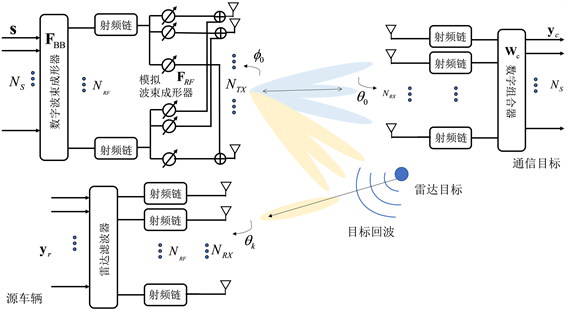Figure 1. Hybrid beamforming framework for millimeter-wave large-scale antenna systems

2.2. 接收信号模型

1) 通信接收信号模型：考虑毫米波信道模型 ： ${H}_{\text{c}}=\alpha {a}_{\text{RX}}\left({\theta }_{0}\right){a}_{\text{TX}}^{*}\left({\varphi }_{0}\right)\in {ℂ}^{{N}_{\text{RX}}×{N}_{\text{TX}}}$，其中， $\alpha$ 是复信道增益； ${a}_{\text{TX}}\left({\varphi }_{0}\right)/{a}_{\text{RX}}\left({\theta }_{0}\right)$ 表示发射/接收天线阵列导向矢量。接收信号经过基带组合器 ${W}_{\text{c}}$ 后表示为：

${y}_{\text{c}}={W}_{\text{c}}^{*}{H}_{\text{c}}x+{W}_{\text{c}}^{*}{z}_{\text{c}}={W}_{\text{c}}^{*}{H}_{\text{c}}{F}_{\text{RF}}{F}_{\text{BB}}s+{W}_{\text{c}}^{*}{z}_{\text{c}}$ (1)

${z}_{\text{c}}~\mathcal{C}\mathcal{N}\left(0,{\sigma }_{\text{c}}^{2}{I}_{{N}_{\text{RX}}}\right)$ 是复加性高斯噪声。因此，接收信号与发射信号之间的均方误差定义为：

$\text{MSE}=\mathbb{E}\left[\left(s-{y}_{\text{c}}\right){\left(s-{y}_{\text{c}}\right)}^{*}\right]$ (2)

2) 雷达接收信号模型：假定 ${K}_{t}$ 个目标车辆和 $K-{K}_{t}$ 个多路径散射分量。关于雷达目标的LoS路径的

${y}_{\text{r}}\left[k\right]={W}_{\text{r}}^{*}{H}_{\text{t}}x\left[k\right]+{W}_{\text{r}}^{*}{z}_{\text{cl}}+{W}_{\text{r}}^{*}{z}_{\text{n}}={W}_{\text{r}}^{*}{H}_{\text{t}}{F}_{\text{RF}}{F}_{\text{BB}}s\left[k\right]+{W}_{\text{r}}^{*}{z}_{\text{cl}}+{W}_{\text{r}}^{*}{z}_{\text{n}},$ (3)

${\zeta }_{\text{rad}}={\mathcal{E}}_{\text{s}}{|{W}_{\text{r}}^{*}{H}_{\text{r}}{F}_{\text{RF}}{F}_{\text{BB}}|}^{2}/\left({\sigma }_{\text{cl}}^{2}+{\sigma }_{\text{n}}^{2}\right){W}_{\text{r}}^{*}{W}_{\text{r}}$ (4)

3. 混合波束形成设计

$\mathcal{P}\left\{\begin{array}{l}{\mathrm{min}}_{{W}_{c},{F}_{\text{RF}},{F}_{\text{BB}},W}\mathrm{MSE}\hfill \\ \text{s}\text{.t}\text{.}:{\zeta }_{\text{rad}}\ge {\text{SINR}}_{0},{F}_{\text{RF}}\in {\mathcal{F}}_{\text{RF}},{‖{F}_{\text{RF}}{F}_{\text{BB}}‖}_{F}^{2}={N}_{S}\hfill \end{array}$ (5)

3.1. 关于Wc的子问题及其求解方法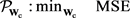(6)

${W}_{\text{c}}={\left[{H}_{\text{c}}{F}_{\text{RF}}{F}_{\text{BB}}{F}_{\text{BB}}^{*}{F}_{\text{RF}}^{*}{H}_{\text{c}}^{*}+{\sigma }_{\text{c}}^{2}{I}_{{N}_{\text{RX}}}\right]}^{-1}{H}_{\text{c}}{F}_{\text{RF}}{F}_{\text{BB}}$ (7)

3.2. 关于Wr的子问题及其求解方法

${W}_{\text{r}}$ 仅仅和雷达SINR约束相关，其最小方差无失真响应(MVDR)问题  和其闭式解为：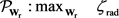(8)

${W}_{\text{r}}={\left[\left({\sigma }_{\text{cl}}^{2}+{\sigma }_{\text{n}}^{2}\right){I}_{{N}_{\text{RX}}}\right]}^{-1}\left({H}_{\text{r}}{F}_{\text{RF}}{F}_{\text{BB}}\right)/\left[{\left({H}_{\text{r}}{F}_{\text{RF}}{F}_{\text{BB}}\right)}^{*}{\left[\left({\sigma }_{\text{cl}}^{2}+{\sigma }_{\text{n}}^{2}\right){I}_{{N}_{\text{RX}}}\right]}^{-1}\left({H}_{\text{r}}{F}_{\text{RF}}{F}_{\text{BB}}\right)\right]$ (9)

3.3. 关于FBB的子问题及其求解方法

${\mathcal{P}}_{{F}_{\text{BB}}}\left\{\begin{array}{l}{\mathrm{min}}_{{F}_{\text{BB}}}\text{MSE}\hfill \\ \text{s}\text{.t}\text{.}:{\zeta }_{\text{rad}}\ge {\text{SINR}}_{0},{‖{F}_{\text{RF}}{F}_{\text{BB}}‖}_{F}^{2}={N}_{S}\hfill \end{array}$ (10)

${F}_{\text{BB}}^{\star }\left(\lambda \right)={\left[{F}_{\text{RF}}^{*}{H}_{\text{c}}^{*}{W}_{\text{c}}{W}_{\text{c}}^{*}{H}_{\text{c}}{F}_{\text{RF}}-\frac{\lambda {\mathcal{E}}_{\text{s}}\left({F}_{\text{RF}}^{*}{H}_{\text{r}}^{*}{W}_{\text{r}}{W}_{\text{r}}^{*}{H}_{\text{r}}{F}_{\text{RF}}\right)}{\left({\sigma }_{\text{cl}}^{2}+{\sigma }_{\text{n}}^{2}\right){W}_{\text{r}}^{*}{W}_{\text{r}}}\right]}^{-1}{F}_{\text{RF}}^{*}{H}_{\text{c}}^{*}{W}_{\text{c}}$ (11)

3.4. 关于FRF的子问题及其求解方法

${\mathcal{P}}_{{F}_{\text{RF}}}\left\{\begin{array}{l}{\mathrm{min}}_{{F}_{\text{RF}}}\text{MSE}\hfill \\ \text{s}\text{.t}\text{.}:{\zeta }_{\text{rad}}\ge {\text{SINR}}_{0},{F}_{\text{RF}}\in {\mathcal{F}}_{\text{RF}},{‖{F}_{\text{RF}}{F}_{\text{BB}}‖}_{F}^{2}={N}_{S}\hfill \end{array}$ (12)

3.5. 四个变量的联合设计

4. 仿真实验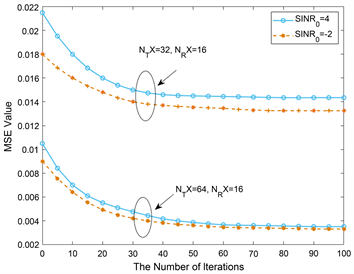(a)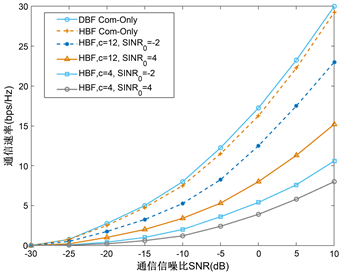(b)

Figure 2. (a) Convergence of AIOM; (b) The realizable rate of vehicle communication under different radar constraints ( ${N}_{\text{TX}}=64$, ${N}_{\text{RX}}=16$ )(a)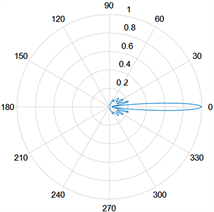(b)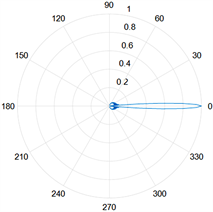(c)

Figure 3. A single beam formed by a hybrid beam. (a) ${N}_{\text{TX}}=16$ ; (b) ${N}_{\text{TX}}=32$ ; (c) ${N}_{\text{TX}}=64$(a)(b)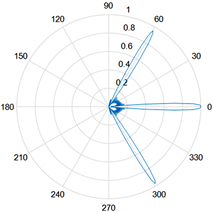(c)

Figure 4. Hybrid beamforming multibeam. (a) ${N}_{\text{TX}}=16$ ; (b) ${N}_{\text{TX}}=32$ ; (c) ${N}_{\text{TX}}=64$

5. 结论

 Liu, F., Masouros, C., Petropulu, A.P., et al. (2020) Joint Radar and Communication Design: Applications, State-of-the-Art, and the Road Ahead. IEEE Transactions on Communications, 68, 3834-3862.

 Guizani, Z. and Hamdi, N. (2016) mmWave E-Band D2D Communications for 5G-Underlay Networks: Effect of Power Allocation on D2D and Cellular Users Throughputs. 2016 IEEE Symposium on Computers and Communication, Messina, 27-30 June 2016, 114-118.
https://doi.org/10.1109/ISCC.2016.7543724

 Chen, Z.M., Cao, Z.X., et al. (2018) DoA and DoD Estimation and Hybrid Beamforming for Radar-Aided mmWave MIMO Vehicular Communication Systems. Elec-tronics, 7, 40.
https://doi.org/10.3390/electronics7030040

 Sohrabi, F. and Wei, Y. (2016) Hybrid Digital and Analog Beamforming Design for Large-Scale Antenna Arrays. IEEE Journal of Selected Topics in Signal Processing, 10, 501-513.
https://doi.org/10.1109/JSTSP.2016.2520912

 Sohail, P., Mysore, B.N., Christos, M., et al. (2018) Phase Shifters vs Switches: An Energy Efficiency Perspective on Hybrid Beamforming. IEEE Wireless Communications Letters, 8, 13-16.

 Qian, J., Lops, M., Zheng, L., et al. (2018) Joint System Design for Coexistence of MIMO Radar and MIMO Communication. IEEE Transactions on Signal Processing, 66, 3504-3519.
https://doi.org/10.1109/TSP.2018.2831624

 Liu, F., Masouros, C., Li, A., et al. (2019) MU-MIMO Commu-nications with MIMO Radar: From Co-Existence to Joint Transmission. IEEE Transactions on Wireless Communica-tions, 17, 2755-2770.
https://doi.org/10.1109/TWC.2018.2803045

 Seel, T., Schauer, T. and Raisch, J. (2017) Monotonic Conver-gence of Iterative Learning Control Systems with Variable Pass Length. International Journal of Control, 90, 393-406.
https://doi.org/10.1080/00207179.2016.1183172

Top